# Full-Form Transformer，The Ultimate Guide

Because of its widespread usage, the MVA full form transformer may be familiar to you if you’re looking to buy one. Megavolt amperes, or M.V.A., is a technical term. Appearance power is measured using this unit. This illustrates that the power will be connected with the current and voltage of a specific circuit.

When describing the overall amount of electrical power in a system, the terms “true power” and “reactive power” must be considered. Additionally, the average rating is often utilized by electrical firms during installations like transformers, substations, and other electrical equipment. MVA values are derived chiefly from other units of measurement, such as kVA or kilovolt amperes.

But how good is an MVA full form transformer? All due to DAELIM, you will discover the solution to this question, its calculation, and many more related to this.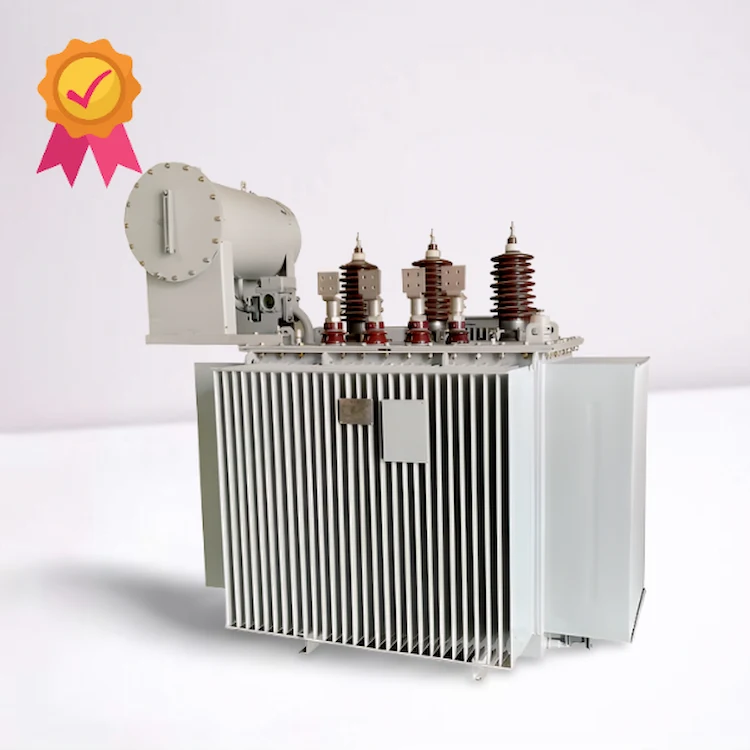We can provide you single phase and three phase pad mounted transformer

#### Dry-type Transformer

Type：Cast resin; Rated Capacity: Up to 25MVA; Rated Voltage: Up to 36KV;

#### Pole Transformer

TypeCSP type Frequency: 50/60Hz; Rated Power: 5~167kva

#### Oil immersed transformer

Frequency: 50/60Hz Rated voltage:10kv, 20kv,30kv Rated Power: 400~2500kva

## Why Daelim is Expert in Transformer Manufacturing

DAELIM has been a firm involved in designing, manufacturing, and manufacturing high-quality electrical equipment for more than 15 years. This enables DAELIM to deliver customers different standards, cutting-edge service, and speed with our expert customization talents.

For the benefit of our clients on six different continents, we at DAELIM have built a company, experience, and technology that others may learn from. Once our clients have created a pooled connection, they are all long-term trusted customers, as we always insist on supplying you with our finest goods and services.

## What is Full Form?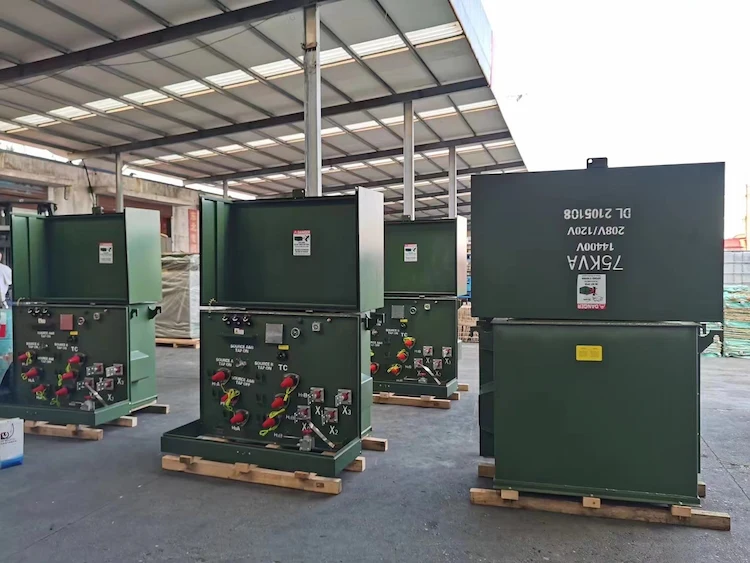A full form, or as some people call it, “full-load,” signifies when the transformer is running at the maximum permitted secondary current. Winding wire size (ampacity) and transformer cooling technique play a role in determining this operating point.

A SPICE transformer simulation, as an example, compares the output voltage with a 1 k-ohm load against a 200 Ohms load, assuming that the 200 Ohms is the “full load.”

##### Get the lastest daelim transformer product cataloge now

In computing an MVA full form-transformer current, you may utilize two techniques: the power calculation method or turns ratio method.

### Power calculation method

Power equations may be categorized into two sorts. The first is the single-phase calculation, and the second is the three-phase calculation.

If the intake of the transformer contains a single-phase (R or Y or B) and neutral (N) indicates, such transformers are termed a single-phase transformer. Meanwhile, when a transformer has three-phase input, it implies that it is termed a three-phase transformer.

### Single-phase transformer current calculations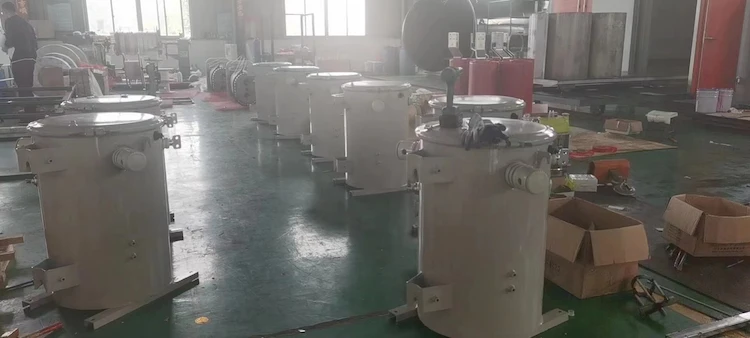To calculate the load current of a single-phase MVA full form transformer, multiply the kVA transformer rating by the primary or secondary voltage V(P-V) or V(S-V) in volts. This yields the I(A) in amps. Full load current is typically solved as I(A) = S(kVA) *1000 / V(V) (V). Yet, the calculation will follow when the transformer’s rate is in MVA. I(A) = S(MVA) *1000000 / V.

### Three-phase transformer current calculations

If you multiply the line-to-line voltage by three, you get the entire load current I(A) in amps, which is the same as 1000 times the transformer rating (S(kVA)). The formula should be I(A) = S(kVA) *1000 / (1.732 * V(V)).

However, when you take the phase to neutral voltage V(L-N) in Volts, it implies the current formula should be I(A) = S(kVA) *1000 / (3 * V(L-N)). As a result, I(P-A) in Amps will be used to calculate primary current, and the formula will be I(P-A) = S(kVA) *1000 / (1.732 * V(P-V))

### Turns ratio approach

The secondary current in Amps is equivalent to the primary current when comparing the primary voltage V(P-V) to the secondary voltage V(S-V) in volts. Generally, the connection may be expressed as, (V(P-V)/V(S-V)) = (I(S-A)/I(P-A)) = (N(P)/ N(S) (S), wherein Np = Primary Turns; Ns = Secondary Turns.

## kVA Full Form Transformer Rating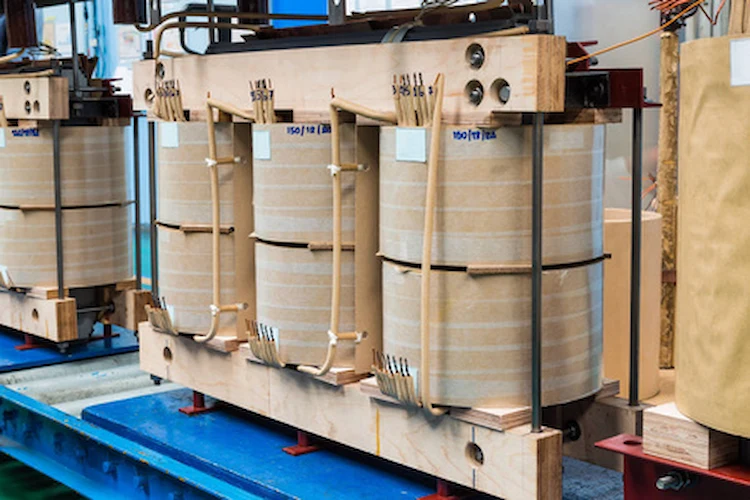KVA, or VA, not kilowatts, is the unit of measurement for transformers. It should be remembered that all-electric loads, including motors, lights, heaters, etc., are all rated in kW, while transformers and generators are rated in kVA.

So, here’s how to calculate kVA Full Form Transformer Rating for your MVA Full Form Transformer.

To know the full load current, you can divide the KVA by the Volts, divided by the product of 1.732 and 1000. You can also use the formula A = KVA ÷ V ÷ 1.732 x 1000. It is where KVA is power in KVA, V is voltage, and A is current in amperes.

Here’s a shortlist of full-load current ratings for general purposes with 240 volts.

2 KVA = 5.6 amps
3 KVA = 8.3 amps
5 KVA = 13.9 amps
6 KVA = 16.7 amps
112.5 KVA = 312.3 amps
750 KVA = 2081.9 amps

However, if you are asked to calculate KVA, you can multiply the volts by the amps and then it to 1.732. The product will then be divided by 1000. In short, you can use the formula KVA = Volts x Amps x 1.732 ÷ 1000 as your formula.

Here’s a shortlist of some samples for drive isolation with a voltage of 230.

7.5 KVA = 18.8 amps
11 KVA = 27.6 amps
14 KVA = 35.1 amps
118 KVA = 296.2 amps
220 KVA = 552.3 amps
440 KVA = 1104.5 amps

In any case, if you need to know the amperes for a single-phase full load current, you can divide the KVS by the volts multiplied by 1000. In short, Amps = KVA ÷ Volts x 1000. But, if KVA is asked to be calculated, you can divide the products of volts and amps by 1000 or KVA = Volts x Amps ÷ 1000.

Here’s a shortlist of amps depending on the KVA and a 120 voltage.

10 KVA = 83.3 amp
37.5 KVA = 312.5 amps
75 KVA = 625.0 amps
167 KVA = 1391.7 amps
333 KVA = 2775.0 amps
500 KVA = 4166.7 amps

## Transformers As Mining Power Supply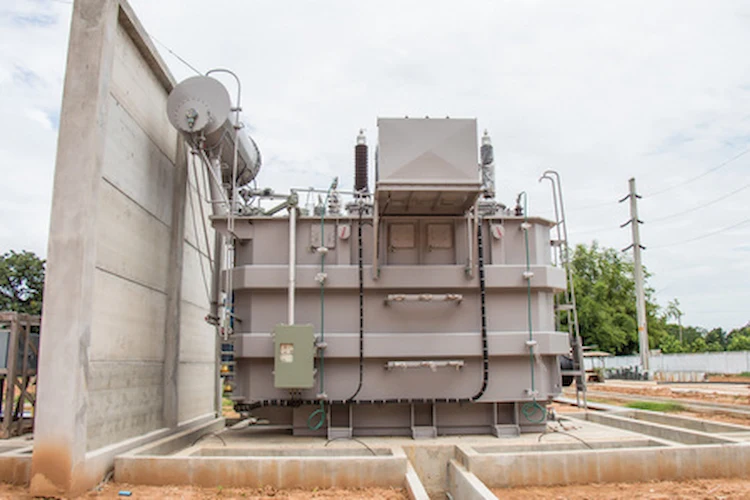The transformer is the first part of your mining power supply that you’ll want to install. Typically, the utility provider is responsible for providing this transformer. However, the customer has the option of offering their own.

Transformers take the medium voltage from a substation and transform it into an applicable low voltage utilized by miners as a Bitcoin mining power system. The ideal way to use transformers as a crypto power source is 1000kVA, 2000kVA, or 5000kVA increments.

In addition, these transformers, which are utilized as a source of power for mining, more significant than this, might incur extra expenditures on the facility’s breaker panels and switchgear. It is typically cheaper to acquire numerous 1000kVA or 2000kVA breaker panels than to produce one huge one.

## What is a voltage drop?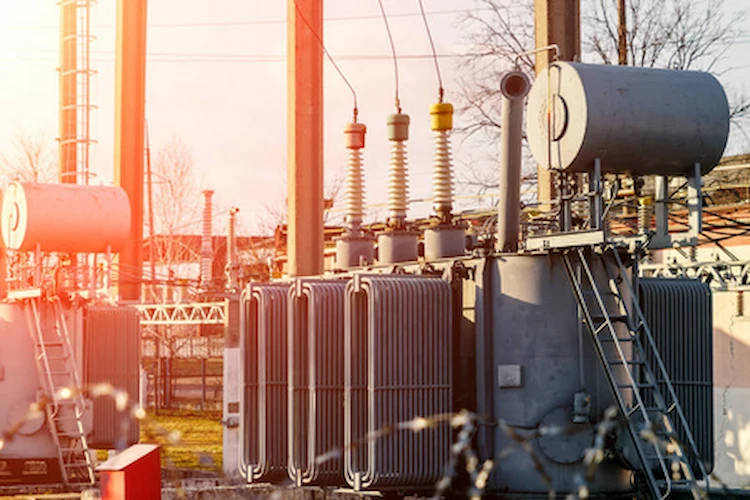Voltage regulation is another term for the transformer voltage drop due to increased load resistance. It measures the transformer’s secondary winding/voltage load’s decrease. So, if there are losses in the I2R, it can significantly impact transformer voltage drop.

## What is the difference between a full-load current, rated current & nominal current?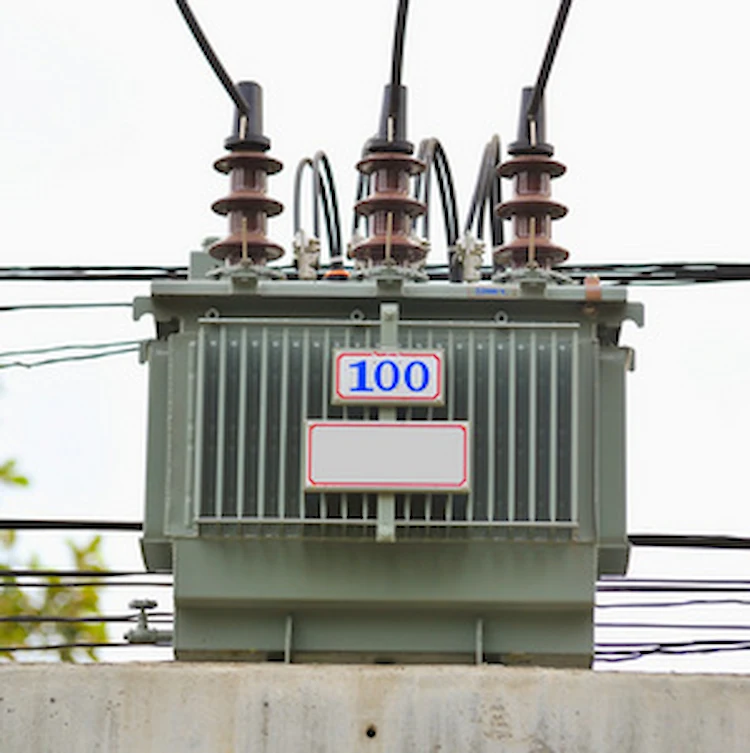Usually, the distinction is in the vocabulary and definitely in specific applications. The full load current is the maximum amount of current that can flow through a piece of equipment when fully loaded under the stipulated parameters.

Rated current is the current listed on the nameplate of the instrument as determined by the manufacturer. A machine’s rated current is also its maximum safe operating current. On the other hand, the nominal current is the maximum current under nominal or rated voltage and power circumstances, primarily the same as the rated current.

All of them symbolize the same thing in most situations. Occasionally, though, the peak current in an ac application may be equal to the whole load current. While the rated current reflects the RMS current at its most significant value.

##### To Know all about the Electric Pole Transformer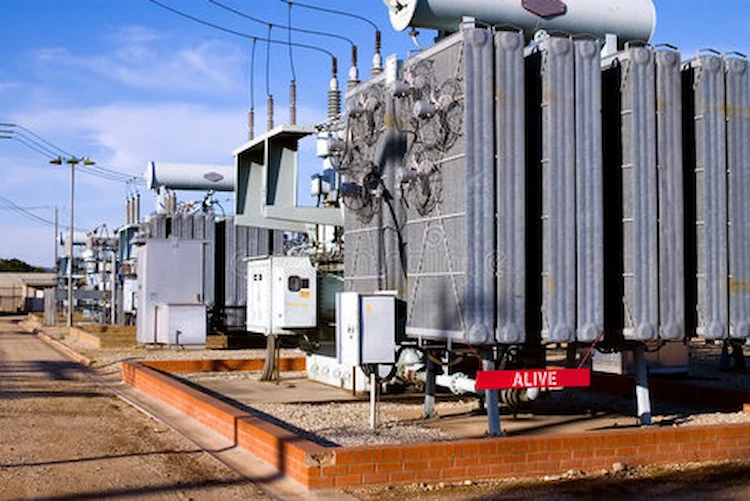The amount of power absorbed by a transformer while it is not connected to any load but still needs some electric current is known as no-load current. Generally, the secondary winding of a transformer in no-load mode is open-circuited.

In a no-load condition, no current can flow on the transformer’s secondary side. If it is connected to a power source, however, a little current, known as a no-load current, will flow through the primary winding. The no-load present value of a transformer varies between 2% and 8% of its maximum load current.

## Takeaway

An MVA Full Form Transformer is vital in preserving some crypto power to lessen the carbon footprint. In fact, you can use a 1 MVA transformer amp to transfer electrical energy between the generator and the principal distribution. As a result, you’ll save money while also accomplishing your role to save the environment.

### 10 kv Oil Filled Transformer

Fault Diagnosis and Analysis of 10 kv Oil Filled Transformer 10 kv Oil filled transformer